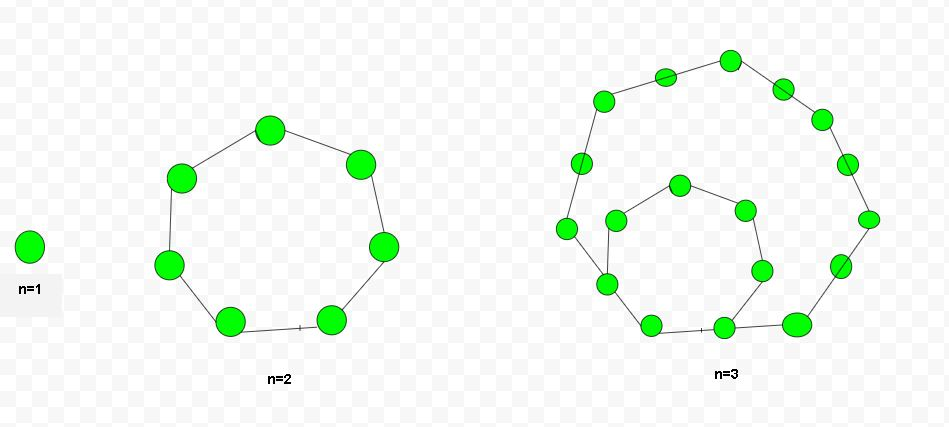# Heptagonal number

Given a number n, the task is to find Nth heptagonal number. A Heptagonal number represents heptagon and belongs to a figurative number. Heptagonal has seven angles, seven vertices, and seven-sided polygon.

Examples :

Input : 2
Output :7

Input :15
Output :540Few Heptagonal numbers are :
1, 7, 18, 34, 55, 81, 112, 148, 189, 235………..

A formula to calculate Nth Heptagonal number:## C++

 // C++ program to find the  // nth Heptagonal number  #include  using namespace std;     // Function to return Nth Heptagonal  // number  int heptagonalNumber(int n)  {      return ((5 * n * n) - (3 * n)) / 2;  }     // Drivers Code  int main()  {         int n = 2;      cout << heptagonalNumber(n) << endl;      n = 15;      cout << heptagonalNumber(n) << endl;         return 0;  }

## Java

 // Java program to find the  // nth Heptagonal number  import java.io.*;     class GFG   {  // Function to return   // Nth Heptagonal number  static int heptagonalNumber(int n)  {      return ((5 * n * n) - (3 * n)) / 2;  }     // Driver Code  public static void main (String[] args)   {      int n = 2;      System.out.println(heptagonalNumber(n));      n = 15;      System.out.println(heptagonalNumber(n));  }  }     // This code is contributed by anuj_67.

## Python3

 # Program to find nth   # Heptagonal number     # Function to find   # nth Heptagonal number  def heptagonalNumber(n) :             # Formula to calculate       # nth Heptagonal number      return ((5 * n * n) -              (3 * n)) // 2    # Driver Code  if __name__ == '__main__' :      n = 2     print(heptagonalNumber(n))      n = 15     print(heptagonalNumber(n))                     # This code is contributed  # by ajit

## C#

 // C# program to find the  // nth Heptagonal number  using System;     class GFG   {  // Function to return   // Nth Heptagonal number  static int heptagonalNumber(int n)  {      return ((5 * n * n) -               (3 * n)) / 2;  }     // Driver Code  public static void Main ()   {      int n = 2;      Console.WriteLine(heptagonalNumber(n));      n = 15;      Console.WriteLine(heptagonalNumber(n));  }  }     // This code is contributed by anuj_67.

## PHP

 

Output :

7
540


My Personal Notes arrow_drop_upCheck out this Author's contributed articles.

If you like GeeksforGeeks and would like to contribute, you can also write an article using contribute.geeksforgeeks.org or mail your article to contribute@geeksforgeeks.org. See your article appearing on the GeeksforGeeks main page and help other Geeks.

Please Improve this article if you find anything incorrect by clicking on the "Improve Article" button below.

Improved By : vt_m, jit_t